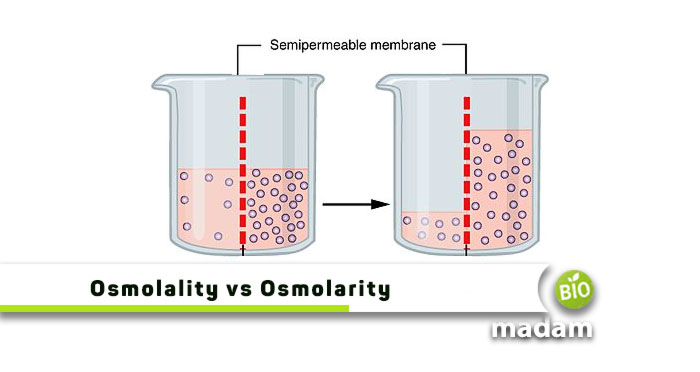# Difference Between Osmolarity And OsmolalityThe terms osmolarity and osmolality are interconnected as both relate to osmotic pressure. This pressure is significant in physics to prevent osmosis. Experts stop a pure solution from transferring to an impure solution, having solutes by applying osmotic pressure. Osmolarity and osmolality particularly define osmotic pressure by measuring it in terms of osmoles.

The main difference between osmolarity and osmolality lies in its measurement. Osmolarity denotes the number of solute particles in one liter of the solvent, just like the molar concentrations. On the other hand, osmolality refers to several solute particles per one kilogram of the solvent, i.e., the number of particles/weight. There are pretty clear distinguishing features of the two, but before that, let’s have a look at them individually.

## Explain Osmolarity

The specific concentration of an osmotic solution is called osmolarity that we represent in osmoles. In simpler words, this term expresses the concentration of a solution in an osmotic activity. We utilize the concept of osmolarity in medical conditions to diagnose various health issues, such as examining dissolved particles in urine. Osmolarity is a complex term, which is quite challenging to calculate. That’s because it depends on changing the volume of solute in a solution (that will also change).

Furthermore, osmolarity is independent of the type of compounds in a solution. Instead, it just analyzes the number of particles added. What affects osmolarity are:

• Changes in the solvent of a solution will change the overall calculations.
• Changes in pressure and temperature directly affect the solubility of compounds.

## Explain Osmolality

This term connects to the number of particles added to a fluid/solution. In general words, osmolality describes this term connects to the number of particles added to a fluid/solution. In general words, osmolality describes the osmotic pressure of a solution where solutes are dissolved in a kilogram of solvent. Like osmolarity, osmolality also helps identify different medical situations, such as dehydration, diabetes, etc. Experts experiment with these diseases where we observe the serum through osmolality, called Plasma Osmolality. Moreover, you can also calculate the concentrations of numerous substances, including urea, potassium, chloride, and glucose.

The center of principle in osmolality is mass which usually doesn’t depend on temperature and pressure. Hence, both these parameters cannot change the osmolality of a solution. Instead, osmolality highly relies on the change in water concentration in a solution.

Despite a few differences, these terms are somehow similar and interlinked too. Both the terms are numerically equal when calculating for highly dilute solutions. Furthermore, a set difference between osmolarity and osmolality is called the Osmolar Gap. It helps as a screening tool to determine different toxins.

## Differences between Osmolarity and Osmolality

Let us now try to tackle and understand some significant differences between the two and how they might affect different properties.

### Units

The units of osmolarity and osmolality are in correspondence to their definitions. We already have mentioned the term, osmole, above which is used in both concepts’ SI unit. Osmole generally has an abbreviation, “Osm.”

Hence, the units representing osmolarity are osmoles/L or Osm/L. On the contrary, the units for osmolality are osmoles/kg or Osm/kg. Moreover, you can easily convert these generally used units to the ones of your choice for convenient calculations, e.g., milliosmoles or micro osmoles – for describing the physiological concentrations of some solutes.

### Calculation of Osmolarity and Osmolality

Calculating osmolarity and osmolality are very easy, just like calculating the number of moles. If we talk about moles, different amounts of NaCl and CaCl­2 will make their one molar solutions corresponding to their molecular weights. But when discussing osmoles, it hinges on how many ions a molecule can contribute, which then multiplies to the molar concentration for desired osmoles. Let us understand this with simple examples and calculations.

#### For Osmolarity

We cannot claim one molar solution of NaCl (molecular weight of NaCl / Liter) to be one osmole of the same NaCl. That is because, in water, NaCl dissociates to form 2 types of ions, i.e., Na+ and Cl-. Therefore the solution would have 1 mole of Na+ and I mole of Cl-, adding together to make two osmoles.

#### For Osmolality

Now let’s comprehend how to calculate osmolality. If we take a sample of NaCl with a contribution of two osmoles per solution, logically, the amount of that sample per kilogram solution would be the osmolality. For instance, adjusting the volume of 1 molar NaCl solution to kilograms would contain two osmoles per kg solvent.

### Temperature Dependency

The change in external or internal temperature brings changes in the volume of solvents accordingly. Let us describe it with a simple example. The volume of a solution increases soon after we increase the solvent (water) temperature in it. Contrastingly, when the temperature decreases, it lowers the overall volume.

Since osmolarity depends on the volume of solutes, temperature changes would change the entire calculation. Thus, affecting osmolarity. Similarly, such solutions also affect other colligative properties.

In contrast, we know that osmolality is measured as solvent per kg, where the change in temperature doesn’t affect mass. Hence, osmolality is independent of the temperature changes. That is why we prefer osmolality over osmolarity for biological systems.

## Final Statement

Despite having visible differences, both osmolarity and osmolality help determine the osmotic pressure. Remember that osmolarity defines a solution by measuring it in osmoles/liter, whereas osmolality does so by osmoles/kilogram.